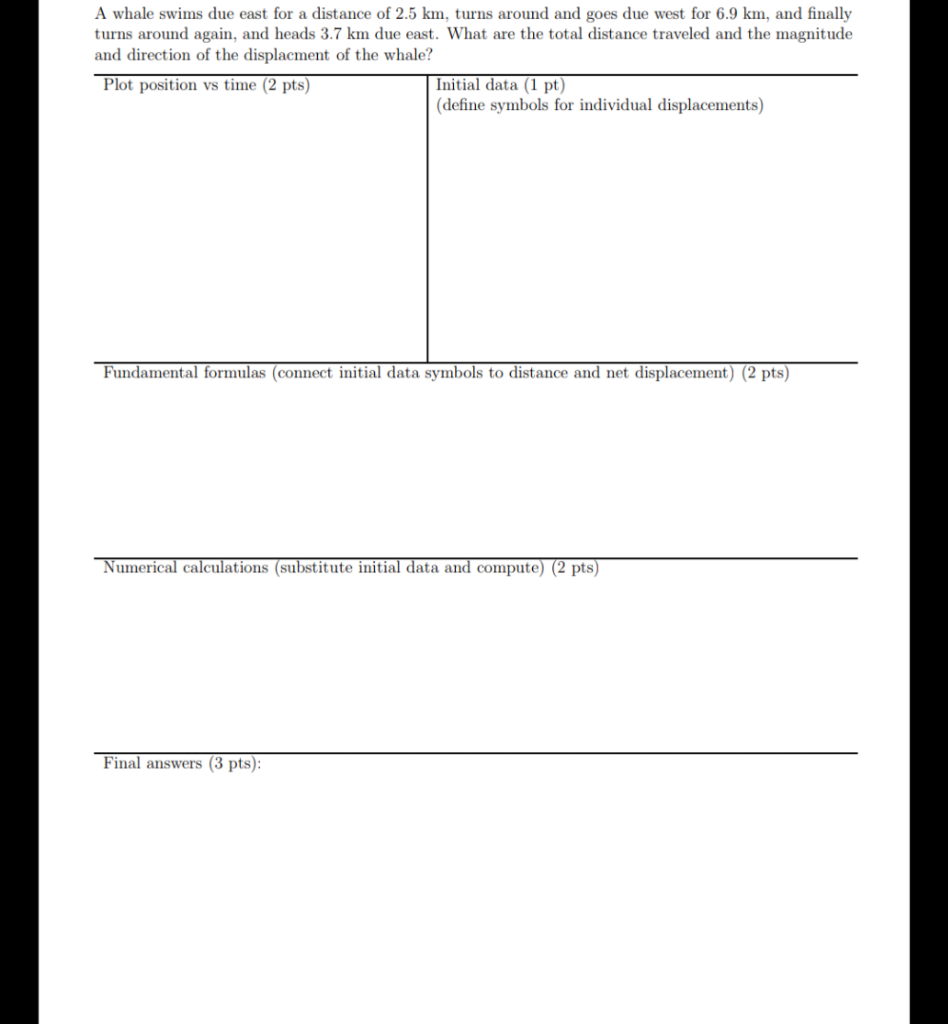# Question A whale swims due east for a distance of 2.5 km, turns around and goes due west for 6.9 km, and finally turns around again, and heads 3.7 km due east. What are the total distance traveled and the magnitude and direction of the displacment of the whale? Plot position vs time (2 pts) Initial data (1 pt) (define symbols for individual displacements) Fundamental formulas (connect initial data symbols to distance and net displacement) (2 pts) Numerical calculations (substitute initial data and compute) (2 pts) Final answers (3 pts):